# Python | Pandas DataFrame.ix[ ]

Python is a great language for doing data analysis, primarily because of the fantastic ecosystem of data-centric Python packages. Pandas is one of those packages and makes importing and analyzing data much easier.

Pandas `DataFrame.ix[ ]` is both Label and Integer based slicing technique. Besides pure label based and integer based, Pandas provides a hybrid method for selections and subsetting the object using the `ix[]` operator. `ix[]` is the most general indexer and will support any of the inputs in `loc[]` and `iloc[]`.

Syntax: DataFrame.ix[ ]

Parameters:
Index Position: Index position of rows in integer or list of integer.
Index label: String or list of string of index label of rows

Returns: Data frame or Series depending on parameters

Code #1:

 `# importing pandas package  ` `import` `pandas as geek ` `   `  `# making data frame from csv file ` `data ``=` `geek.read_csv(``"https://media.geeksforgeeks.org/wp-content/uploads/nba.csv"``)   ` `   `  `# Integer slicing ` `print``(``"Slicing only rows(till index 4):"``) ` `x1 ``=` `data.ix[:``4``, ] ` `print``(x1, ``"\n"``) ` `  `  `print``(``"Slicing rows and columns(rows=4, col 1-4, excluding 4):"``) ` `x2 ``=` `data.ix[:``4``, ``1``:``4``] ` `print``(x2) `

Output :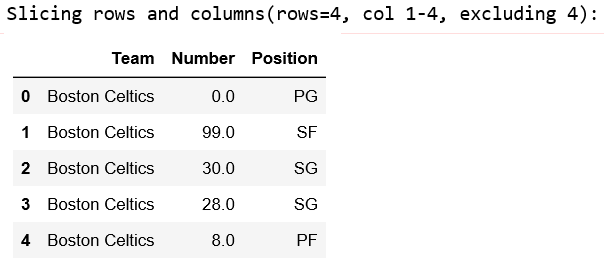Code #2:

 `# importing pandas package  ` `import` `pandas as geek ` `   `  `# making data frame from csv file ` `data ``=` `geek.read_csv(``"nba.csv"``)   ` `   `  `# Index slicing on Height column ` `print``(``"After index slicing:"``) ` `x1 ``=` `data.ix[``10``:``20``, ``'Height'``] ` `print``(x1, ``"\n"``) ` ` `  `# Index slicing on Salary column ` `x2 ``=` `data.ix[``10``:``20``, ``'Salary'``] ` `print``(x2) `

Output: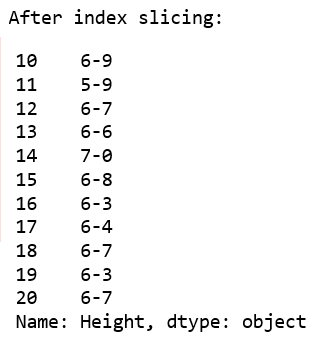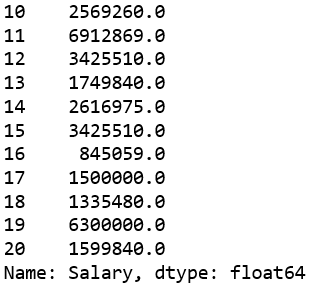Code #3:

 `# importing pandas and numpy ` `import` `pandas as pd ` `import` `numpy as np ` `  `  `df ``=` `pd.DataFrame(np.random.randn(``10``, ``4``), ` `          ``columns ``=` `[``'A'``, ``'B'``, ``'C'``, ``'D'``]) ` ` `  `print``(``"Original DataFrame: \n"` `, df) ` `  `  `# Integer slicing ` `print``(``"\n Slicing only rows:"``) ` `print``(``"--------------------------"``) ` `x1 ``=` `df.ix[:``4``, ] ` `print``(x1) ` `  `  `print``(``"\n Slicing rows and columns:"``) ` `print``(``"----------------------------"``) ` `x2 ``=` `df.ix[:``4``, ``1``:``3``] ` `print``(x2) `

Output :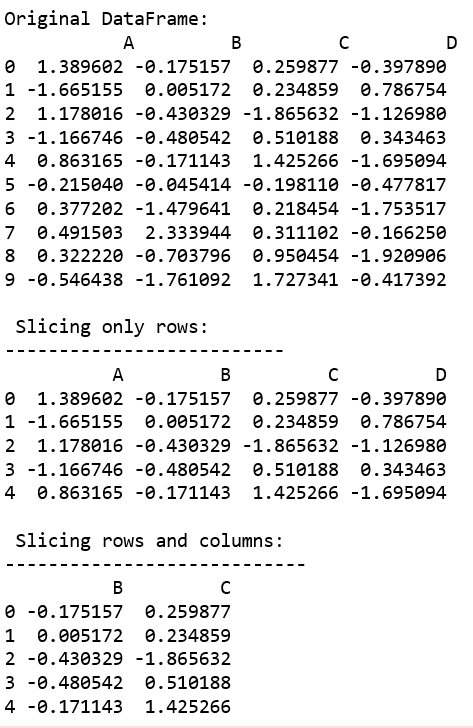Code #4:

 `# importing pandas and numpy ` `import` `pandas as pd ` `import` `numpy as np ` `  `  `df ``=` `pd.DataFrame(np.random.randn(``10``, ``4``), ` `          ``columns ``=` `[``'A'``, ``'B'``, ``'C'``, ``'D'``]) ` ` `  `print``(``"Original DataFrame: \n"` `, df) ` `  `  `# Integer slicing (printing all the rows of column 'A') ` `print``(``"\n After index slicing (On 'A'):"``) ` `print``(``"--------------------------"``) ` `x ``=` `df.ix[:, ``'A'``] ` ` `  `print``(x) `

Output :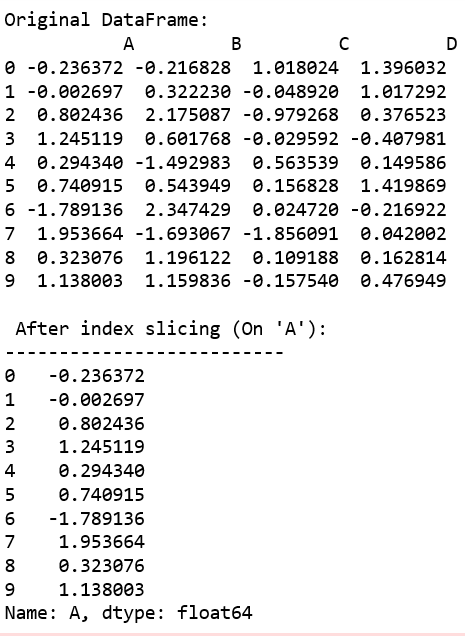My Personal Notes arrow_drop_upCheck out this Author's contributed articles.

If you like GeeksforGeeks and would like to contribute, you can also write an article using contribute.geeksforgeeks.org or mail your article to contribute@geeksforgeeks.org. See your article appearing on the GeeksforGeeks main page and help other Geeks.

Please Improve this article if you find anything incorrect by clicking on the "Improve Article" button below.

Article Tags :

1

Please write to us at contribute@geeksforgeeks.org to report any issue with the above content.